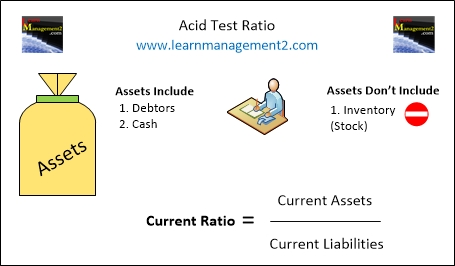# Liquidity Ratios

## Acid Test Ratio

Firms often need to borrow money to invest in the business and help it grow. Debts are not a problem as long as they are managed properly. The Acid Test ratio can be used to find out if a firm is borrowing money sensibly. The Acid Test ratio looks at whether a firm has enough current assets to pay back its short term debt (current liabilities). The Acid Test ratio is different to the Current Ratio because stock is not included in the (value of the) current assets used in the calculation.This diagram shows how to calculate the Acid Test Ratio

### Why is Stock (inventory) Not Included In the Calculation?

Stock (inventory) can not always be converted into cash quickly. Inventory can “go out of date”, get damaged or lose value, so the acid test ratio shows you how many times the business can pay off its current liabilities, if stock is not included as a current asset.

How Do I Calculate the Acid Test Ratio For a Firm

Acid Test Ratio              Current Assets - Stock
=          __________________

Current Liabilities

### Example Calculation

For example if my current assets are £9000 which includes £3000 of stock and my current liabilities are £2000, I would work out my current ratio as follows

Current Assets - Stock                       9000 – 3000                6000
_________________              =          _________      =          _____  = 3

Current Liabilities                                2000                            2000

### What Does the Answer (number 3) in the Example Mean?

In this example I can use my current assets to pay off my current liabilities three times. In other words my current assets are three times the size of my current liabilities.

### How Do I Write The Ratio?

As Liquidity ratios are expressed against 1 my acid test ratio for this example is 3:1.

### Conclusion

We hope you've enjoyed learning about the Acid Test Ratio. We have related Financial Ratio articles for you to read; they are a great way to increase your understanding about Financial Ratios.

Related Articles: | Current Ratio | Liquidity Ratios Overview | Liquidity & Working Capital | Introduction To Financial Ratios